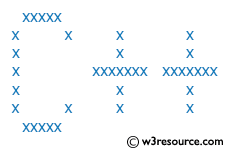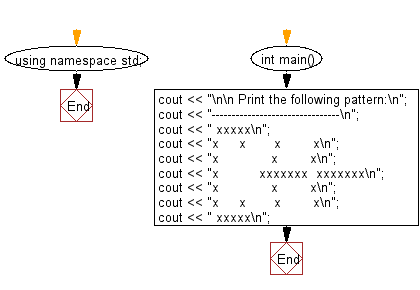﻿ C++ Exercises: Print the following pattern - w3resource

# C++ Exercises: Print the following pattern

## C++ Basic: Exercise-39 with Solution

Write a C++ program to print the following pattern.

Pictorial Presentation:``` xxxxx
x     x       x        x
x             x        x
x          xxxxxxx  xxxxxxx
x             x        x
x     x       x        x
xxxxx
```

Sample Solution:

C++ Code :

``````#include <iostream>
using namespace std;

int main()
{
cout << "\n\n Print the following pattern:\n";
cout << "--------------------------------\n";
cout << " xxxxx\n";
cout << "x     x       x        x\n";
cout << "x             x        x\n";
cout << "x          xxxxxxx  xxxxxxx\n";
cout << "x             x        x\n";
cout << "x     x       x        x\n";
cout << " xxxxx\n";
}
``````

Sample Output:

``` Print the following pattern:
--------------------------------
xxxxx
x     x       x        x
x             x        x
x          xxxxxxx  xxxxxxx
x             x        x
x     x       x        x
xxxxx
```

Flowchart:C++ Code Editor:

What is the difficulty level of this exercise?

﻿

## C++ Programming: Tips of the Day

Why is there no std::stou?

The most pat answer would be that the C library has no corresponding "strtou", and the C++11 string functions are all just thinly veiled wrappers around the C library functions: The std::sto* functions mirror strto*, and the std::to_string functions use sprintf.

Ref: https://bit.ly/3wtz2qA

We are closing our Disqus commenting system for some maintenanace issues. You may write to us at reach[at]yahoo[dot]com or visit us at Facebook